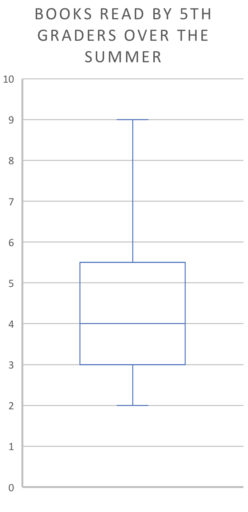We have already talked about what the mean, median, and mode are. But in the examples we discussed previously, we gave you the data and asked you to find the mean/median/mode. But you can also sometimes, to a limited degree, get information about the mean/median/mode just from the graph of the data. For example:

Example 1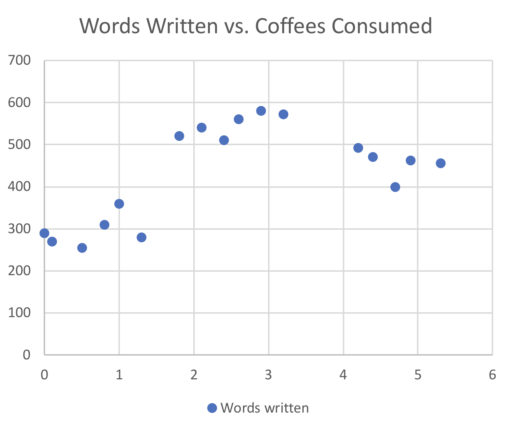Which of the following two quantities is larger?

• Average number of words written
•A. The average number of words written is larger

B.is larger

C. They are equal

D. It cannot be determined from the information given

Now, sometimes you will be asked to manipulate the data/graph given before estimating the mean/median/mode. So, for example:

Example 2Suppose the researchers for the above graph miscounted the number of words each participant wrote. They accidentally multiplied the number of words written by each participant by 2. Fix this error in their data by dividing the observations in the above graph by 2 to get the new average. Now, which of the following two quantities is larger?

• New average of words written
•A. The average number of words written is larger

B.is larger

C. They are equal

D. It cannot be determined from the information given

Now, we can generally find the median from a graph as well:

Example 3Find the median number of words written in the above scatterplot.

And again, we can modify the given data and find the new median:

Example 4

Suppose that, this time, the researchers under-counted the number of words each participant wrote. Find the median of the corrected data, multiplying the number of words written by each participant by 2.

And finally, we could try to find the mode of the above graph. It is somewhat hard to tell whether some of the points are the same on the above graph, so I will cheat and just tell you that there is no mode; every value occurs just once. But on the GRE, rest assured that if the question asks you to find the mode from the graph, the relevant points will be fairly clearly marked. Then, it is just a matter of counting up how many observations each value has (e.g. how many people wrote 500 words; wrote 550; etc.).

Finding quartiles/percentiles

Now, it will not always be possible to find the quartile of a graph. For example, if you are given a circle graph: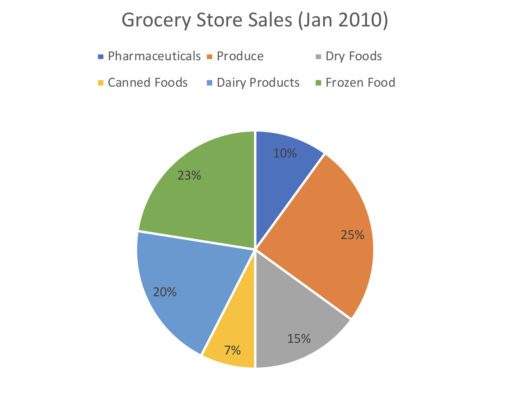and asked to find the various quartiles, the question simply makes no sense. But of course, in a box plot: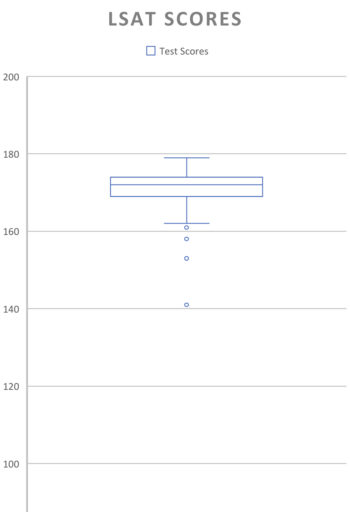the quartiles just correspond to where the lines of the box are.

Now, it is not really feasible to read off, say, what the 96th percentile looks like, just based off of a graph. But some important facts to keep in mind are that: the highest value in your graph will be greater than the 99th percentile (since all of the observations will be less than or equal to that observation’s value). Similarly, the 1st percentile will be greater than the lowest value of the graph. Thus, knowing the maximum and minimum (which you can read off of a graph) can give you some idea of the limits of your percentiles.

Practice Problems

1. In the below histogram, what if anything can we conclude about the median of the data?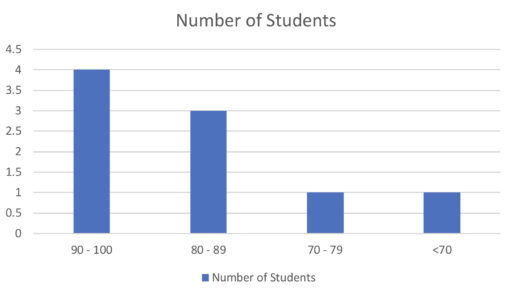2. In the following chart, what can we conclude about the range of the data?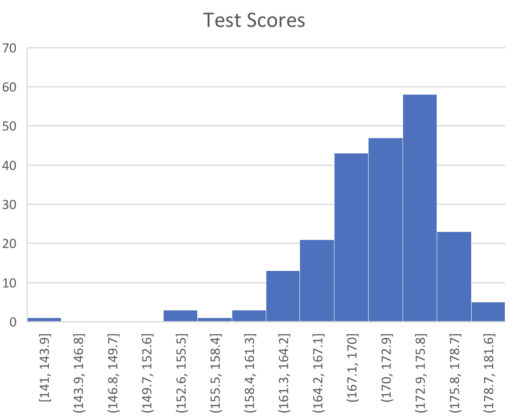3. A new kid, Richard, joins the class. Richard is 4'8. Given that the graph below accurately depicts the heights of Richard's classmates, what is the maximum possible number of classmates that are taller than Richard? What is the minimum possible number?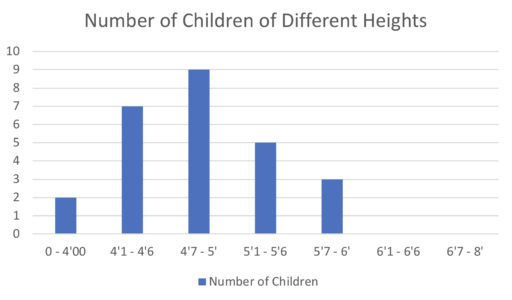4. In the below chart, what if anything can you conclude about the median height of the class?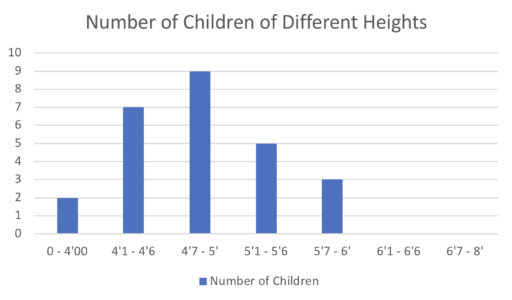5. The following chart represents the books read by the 500 fifth-graders in a school over the summer. Suppose 7 is the 80th percentile of this data. Approximately how many people read between 6 and 7 books? (We assume that people can only read positive integers of books).